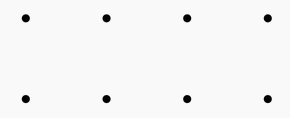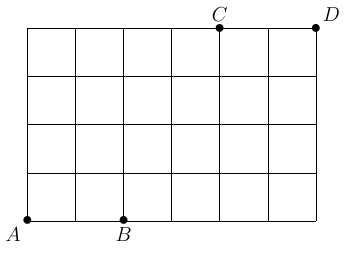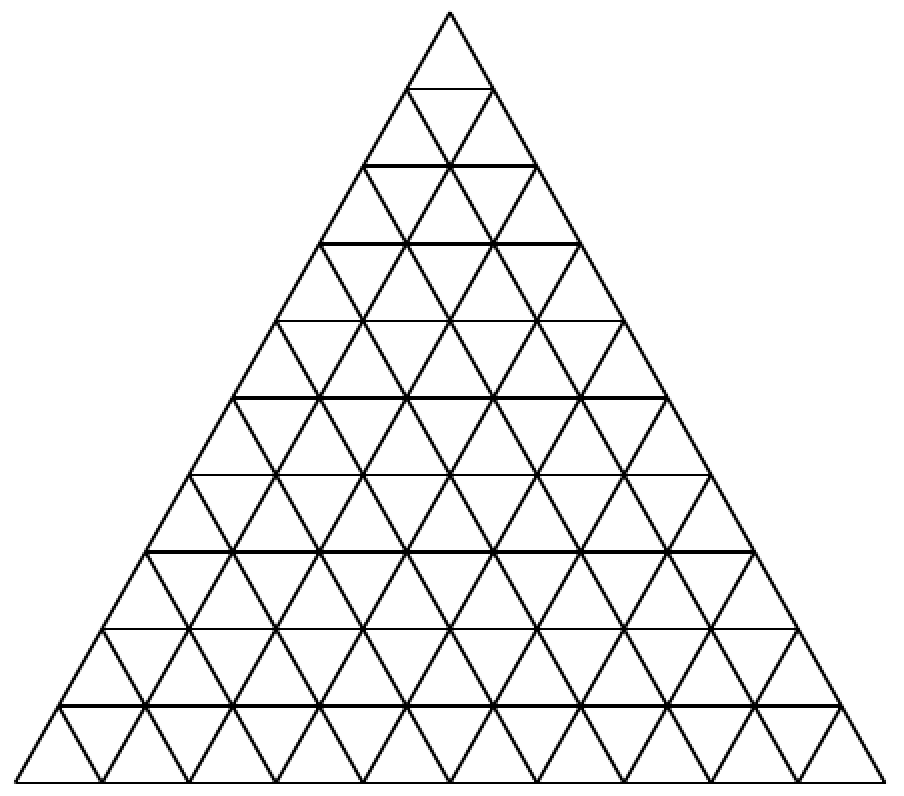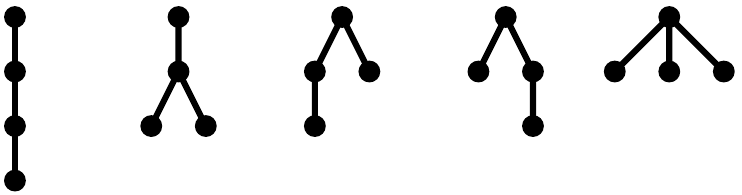###### back to index | new

Ten people form a line, among which two are Chinese and two are Americans. Find the probability that both Chinese will stand in front of both Americans (not necessarily immediately in the front).

Let $A={1,2,3,4}$, and $f$ and $g$ be randomly chosen (not necessarily distinct) functions from $A$ to $A$. Find the probability that the range of $f$ and the range of $g$ are disjoint.

Define an ordered quadruple of integers $(a, b, c, d)$ as interesting if $1 \le a < b < c < d \le 10$, and $a+d>b+c$. How many interesting ordered quadruples are there?

How many non-congruent triangles have vertices at three of the eight points in the array shown below?Six people form a line. $A$ must stand after $B$ (not necessarily immediately after $B$). How many different ways are there to form such a line?

How many fractions in simplest form are there between $0$ and $1$ such that the products of their denominators and numerators equal $20!$?

John walks from point $A$ to $C$ while Mary goes from point $B$ to $D$. Both of them will move along the grid, either right or up, so they take shortest routes. How many different possibilities are there such that their routes do not intersect?Find the number of non-decrease sequences of length $n$ and each element is a non-negative integer not exceeding $d$.

A partition of a positive integer $n$ is to write $n$ as a sum of some positive integers. Let $k$ be a positive integer. Show that the number of partitions of $n$ with exactly $k$ parts equals the number of partitions of $n$ whose largest part is exactly $k$.

A partition of a positive integer $n$ is to write $n$ as a sum of some positive integers.. Show that the number of partitions of a positive integer $n$ into distinct parts is equal to the number of partitions of $n$ where all parts are odd integers.

Find the number of parallelograms in the following equilateral triangle of side length $n$ which is made of some smaller unit equilateral triangles.Let $n$ be a positive integer. Find the number of paths from $(0,0)$ to $(n,n)$ on a $n\times n$ grid using only unit up and right steps so that the path stays in the region $x\ge y$.

Find the number of expressions containing $n$ pairs of parentheses which are correctly matched. For example, when $n=3$, the answer is $5$ because all the valid expressions are $$((())),\ (()()),\ (())(),\ ()(()),\ ()()()$$

A tree is a structure which grows from a single root node. By convention, the root node is usually placed at the top. Then, new nodes, referred as children nodes, can be attached to an existing node, referred as parent node, by edges. The root node has no parent and all other nodes have exactly one parent. A node may have any number of children nodes, including no child at all. No looping chain of nodes is permitted in this structure. For example, there are exactly $5$ different types of trees with $4$ nodes. They are shown below. The goal is to find the number of different types of tree with $n$ nodes where $n$ is a positive integer greater than $1$.Let positive integers $n$ and $k$ satisfy $n\ge 2k$. How many $k$-sided convex polygons are there whose vertices are those of an $n$-sided convex polygon and edges are diagonals of the same $n$-polygon.

Given a convex $n$-polygon, what is the max number of intersection points can its diagonals form? (Vertices do not count.)

Assuming positive integer $n$ satisfies $n\equiv 1\pmod{4}$ and $n > 1$. Let $\mathbb{P}=\{a_1,\ a_2,\ \cdots,\ a_n\}$ be a permutation of $\{1,\ 2,\ \cdots,\ n\}$. If $k_p$ denotes the largest index $k$ associated with $\mathbb{P}$ such that the following inequality holds $$a_1 + a_2 + \cdots + a_k < a_{k+1}+a_{k+2}+\cdots + a_n$$

Find the sum of $k_p$ for all possible $\mathbb{P}$.

Let $\mathbb{S}=\{1,\ 2,\ \cdots,\ 1000\}$ and $\mathbb{A}$ be a subset of $\mathbb{S}$. If the number of elements in $\mathbb{A}$ is $201$ and their sum is a multiple of $5$, then $\mathbb{A}$ is called $\textit{good}$. How many good $\mathbb{A}$ are there?

There are $n \ge 6$ points on a circle, every two points are connected by a line segment. No three diagonals are concurrent. How many triangles are created by these sides and diagonals?

A child builds towers using identically shaped cubes of different colors. How many different towers with a height of $8$ cubes can the child build with $2$ red cubes, $3$ blue cubes, and $4$ green cubes? (One cube will be left out.)

Equally divide each side of a triangle into $n$ parts and then connect these points to draw lines which are parallel to one of the triangle's sides. Find the number of parallelograms created by these lines.

A permutation $\{x_1,\ x_2,\ \cdots,\ x_{2n}\}$ of the set $\{1,\ 2,\ \cdots,\ 2n\}$, where $n$ is a positive integer, is said to have property $P$ if $\mid x_i − x_{i+1}\mid = n$ for at least one $i$ in $\{1,\ 2,\ \cdots,\ 2n − 1\}$. Show that, for each $n$, there are more permutations with property $P$ than without.

Let $S_n$ be the number of non-congruent triangles whose sides' lengths are all integers and circumferences equals $n$. Show that $$S_{2n-1}-S_{2n} = \left\lfloor\frac{n}{6}\right\rfloor\quad\text{or}\quad\left\lfloor\frac{n}{6}\right\rfloor +1$$

where $\lfloor{x}\rfloor$ returns the largest integer not exceeding the real number $x$.

Find the number of ways to divide a convex $n$-sided polygon into $(n-2)$ triangles using non-intersecting diagonals.

Let $x_i\in\{+1,\ -1\}$, $i=1,\ 2,\ \cdots,\ 2n$. If their sum equals $0$ and the following inequality holds for any positive integer $k$ satisfying $1\le k < 2n$: $$x_1+x_2+\cdots + x_k\ge 0$$

Find the number of possible ordered sequence $\{x_1,\ x_2,\ \cdots,\ x_{2n}\}$.